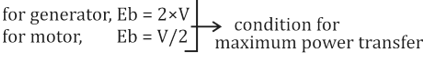Engineering Jobs   »   DFCCIL ELECTRICAL QUIZ

# DFCCIL’21 EE: Daily Practices Quiz 13-Aug-2021

Each question carries 1 mark.
Negative marking: 1/4 mark
Total Questions: 06
Time: 06 min.

Q1. Non-minimum phase transfer function is defined as the transfer function
(a) which has zero in the right-half s-plane
(b)which has zero only in the left-half s-plane
(c) which has poles in the right-half s-plane
(d)which has poles in the left-half s-plane

Q2. JFET is a ______ carrier device.
(a) Minority (Unipolar)
(b) Minority (Bipolar)
(c) Majority (Unipolar)
(d) Majority (Bipolar)

Q3. A SCR (Silicon Controlled Rectifier) is a
(a) Device with 3 junctions
(b) Device with 2 junctions
(c) Device with 1 junction
(d) Device with 4 junctions

Q4. 1’s compliment of 11001010 is
(a) 00110111
(b) 00110101
(c) 11001001
(d) 11001011

Q5. The current in the inductor lags the voltage in a series RLC circuit ___________ resonant frequency.
(a) Above
(b) Below
(c) Equal to
(d) Depends on the circuit

Q6. Condition for maximum power delivered in D.C. Generator is_______:
(a) Back EMF = Supply voltage
(b) Back EMF = (2×Supply voltage)
(c) Back EMF = (Supply voltage/4)
(d) Back EMF = (Supply voltage/9)

SOLUTIONS
S1. Ans.(a)
Sol. non-minimum phase transfer function is defined as the transfer function which has one or more zeros in the right half of s – plane and remaining poles and zeros in the left half of s – plane.
NOTE:
Stability of the system depends on pole location.
Minimum phase transfer function depends on left side poles and zero.

S2. Ans.(c)
Sol. The current flow in the device is due to the majority carriers. In an n-type JFET, it is due to the electrons, and in a p-type JFET- it is due to the holes.
JFET is a unipolar and voltage-controlled device.

S3. Ans.(a)
Sol. SCR stands for Silicon Controlled Rectifier; it is a family of thyristors with the same property as thyristors. It is a semiconductor device with three junctions. They are of two types: npnp and pnpn.

S4. Ans.(b)
Sol. 1’s complement of a binary number can be found out by inverting each bit in the binary number system.

S5. Ans.(a)
Sol. The current in the inductor lags the voltage in a series RLC circuit if a circuit is inductive dominant i.e., ω > ω_0. So, the current in the inductor lags the voltage in a series RLC circuit above the resonant frequency.

S6. Ans.(b)
Sol.Sharing is caring!